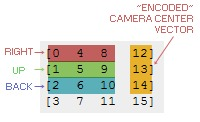To main page | 3dengine.org

# Modelview matrix

Modelview matrix is a matrix that stores right, up and back and translation vectors. It can be thought of as showing where you are in space and how much is your head tilted in 3 directions.See Right-up-back vectors from modelview matrix for explanation.

The Right, Up and Back vectors are 3 numbers that show the directions where the camera is pointing, i.e. right vector: if the vector in camera space is pointing right, what coordinates does it have in world space?

Upper 3x3 matrix of modelview matrix (0,4,8,1,5,9,2,6,10) is called rotation matrix.

Elements (12,13,14) are called translation vector.

The bottom row (3,7,11,15) is always (or at least it should be) = [0,0,0,1].

This matrix should be affected by glRotate, glTranslate calls, i.e. call glMatrixMode(GL_MODELVIEW) before those.

Contrarily: glPerspective, glFrustum should be done on projection matrix.

## PyOpenGL

Getting:
```model = glGetDoublev(GL_MODELVIEW_MATRIX)
```
Setting:
```glMatrixMode(GL_MODELVIEW)
```

## C++

Getting:
```GLdouble model;
glGetDoublev(GL_MODELVIEW_MATRIX, model);
```
Setting:
```GLdouble model;
....
glMatrixMode(GL_MODELVIEW);